## Kinematics in One Dimension

Kinematics analyzes the positions and motions of objects as a function of time, without regard to the causes of motion. It involves the relationships between the quantities displacement (d), velocity (v), acceleration (a), and time (t). The first three of these quantities are vectors.

## Definition of a vector

A vector is a physical quantity with direction as well as magnitude, for example, velocity or force. In contrast, a quantity that has only magnitude and no direction, such as temperature or time, is called a scalar. A vector is commonly denoted by an arrow drawn with a length proportional to the given magnitude of the physical quantity and with direction shown by the orientation of the head of the arrow.

## Displacement and velocity

Imagine that a car begins traveling along a road after starting from a specific sign post. To know the exact position of the car after it has traveled a given distance, it is necessary to know not only the miles it traveled but also its heading. The displacement, defined as the change in position of the object, is a vector with the magnitude as a distance, such as 10 miles, and a direction, such as east. Velocity is a vector expression with a magnitude equal to the speed traveled and with an indicated direction of motion. For motion defined on a number line, the direction is specified by a positive or negative sign. Average velocity is mathematically defined asNote that displacement (distance from starting position) is not the same as distance traveled. If a car travels one mile east and then returns one mile west, to the same position, the total displacement is zero and so is the average velocity over this time period. Displacement is measured in units of length, such as meters or kilometers, and velocity is measured in units of length per time, such as meters/second (meters per second).

## Average acceleration

Acceleration, defined as the rate of change of velocity, is given by the following equation:Acceleration units are expressed as length per time divided by time such as meters/second/second or in abbreviated form as m/s 2.

## Graphical interpretations of displacement, velocity, and acceleration

The distance versus time graph in Figure shows the progress of a person (I) standing still, (II) walking with a constant velocity, and (III) walking with a slower constant velocity. The slope of the line yields the speed. For example, the speed in segment II is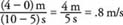Figure 1

Motion of a walking person.

Each segment in the velocity versus time graph in Figure depicts a different motion of a bicycle: (I) increasing velocity, (II) constant velocity, (III) decreasing velocity, and (IV) velocity in a direction opposite the initial direction (negative). The area between the curve and the time axis represents the distance traveled. For example, the distance traveled during segment I is equal to the area of the triangle with height 15 and base 10. Because the area of a triangle is (1/2)(base)(height), then (1/2)(15 m/s)(10 s) = 75 m. The magnitude of acceleration equals the calculated slope. The acceleration calculation for segment III is (−15 m/s)/(10 s) = −1.5 m/s/s or −1.5 m/s 2.Figure 2

Accelerating motion of a bicycle

The more realistic distance‐versus‐time curve in Figure (a) illustrates gradual changes in the motion of a moving car. The speed is nearly constant in the first 2 seconds, as can be seen by the nearly constant slope of the line; however, between 2 and 4 seconds, the speed is steadily decreasing and the instantaneous velocity describes how fast the object is moving at a given instant.Figure 3

Motion of a car: (a) distance, (b) velocity, and (c) acceleration change in time.

Instantaneous velocity can be read on an odometer in the car. It is calculated from a graph as the slope of a tangent to the curve at the specified time. The slope of the line sketched at 4 seconds is 6 m/s. Figure (b) is a sketch of the velocity‐versus‐time graph constructed from the slopes of the distance‐versus‐time curve. In like fashion, the instantaneous acceleration is found from the slope of a tangent to the velocity‐versus‐time curve at a given time. The instantaneous acceleration‐versus‐time graph in Figure (c) is the sketch of the slopes of the velocity‐versus‐time graph of Figure (b). With the vertical arrangement shown, it is easy to compute the displacement, velocity, and acceleration of a moving object at the same time.

For example, at time t = 10 s, the displacement is 47 m, the velocity is −5 m/s, and the acceleration is −5 m/s 2.

## Definitions of instantaneous velocity and instantaneous acceleration

The instantaneous velocity, by definition, is the limit of the average velocity as the measured time interval is made smaller and smaller. In formal terms,. The notation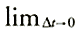means the ratio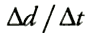is evaluated as the time interval approaches zero. Similarly, instantaneous acceleration is defined as the limit of the average acceleration as the time interval becomes infinitesimally short. That is,.

## Motion with constant acceleration

When an object moves with constant acceleration, the velocity increases or decreases at the same rate throughout the motion. The average acceleration equals the instantaneous acceleration when the acceleration is constant. A negative acceleration can indicate either of two conditions:

• Case 1: The object has a decreasing velocity in the positive direction.
• Case 2: The object has an increasing velocity in the negative direction.

For example, a ball tossed up will be under the influence of a negative (downward) acceleration due to gravity. Its velocity will decrease while it travels upward (case 1); then, after reaching its highest point, the velocity will increase downward as the object returns to earth (case 2).

Using v o (velocity at the beginning of time elapsed), v f (velocity at the end of the time elapsed), and t for time, the constant acceleration is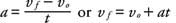(1)

Substituting the average velocity as the arithmetic average of the original and final velocities v avg = ( v o + v f )/2 into the relationship between distance and average velocity d = ( v avg)( t) yields.(2)

Substitute v f from Equation 1 into Equation 2 to obtain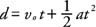(3)

Finally, substitute the value of t from Equation 1 into Equation 2 for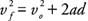(4)

These four equations relate v o , v f , t, a, and d. Note that each equation has a different set of four of these five quantities. Table summarizes the equations for motion in a straight line under constant acceleration.A special case of constant acceleration occurs for an object under the influence of gravity. If an object is thrown vertically upward or dropped, the acceleration due to gravity of −9.8 m/s 2 is substituted in the above equations to find the relationships among velocity, distance, and time.